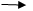15 October, 2020

Ratings

#### Related Blogs

int a = 10; b = 20;,c;

C= a + b-30  (Addition)

String S1 = ‘ABC’, S2 = ‘XYZ’,S3;

S3 = S1+S2Abc xyz  (concatenation)

Assume that test is a class and t1,t2,t3 are objects then

T3 = t1+t2

gives an error ‘+’ operator is developed to use with numbers and string only BUT NOT WITH OBJECTS.

Operator overloading is a concept of extending the functionality of an Existing operator.

All the operators are overloadable except those contains a.

These core tutorials will help you to learn the fundamentals of .NET. For an in-depth understanding and practical experience, explore Online ".NET Training"

That is the following operators are not overloadable.member Access operator

:: Ternary or conditional operator

:: property access operator

:   Inheritance operator

An overloaded operator must be declared as static.

Operator is a keyword.

Public static return type  operator + (args)

{

Logic;

}

Class EMP {

Private int Sal;

.

.

.

.

}

E1Sal (5000) E2Sal (8000) E3Sal (2700)

E1+E2+E3 = EMP(Data type)

Open windows forms Application project à Place a buttonCode in GD

``````Class EMP

{

Private int Sal;

Public EMP (int x  )

// constructor

{

Sal = X;

}

Public EMP ()

// Default constructor

{}

Public void Print ()

{

Message Box. Show (Sal);

}

//overloading  + public static EMP operator  +  (EMP X , EMP y)

{

EMP t = new EMP ();

Sal = X. Sal + Y. Sal;

return t;

}

}// EMP class

Code for Button 1_click

{

EMP e1 = new EMP (5000);

EMP e2 = new EMP (8000);

EMP e3 = new EMP (5000);

EMP total  = new EMP ();

Total = e1 +e2+e3 ;

Total .Print ();

}  ``````